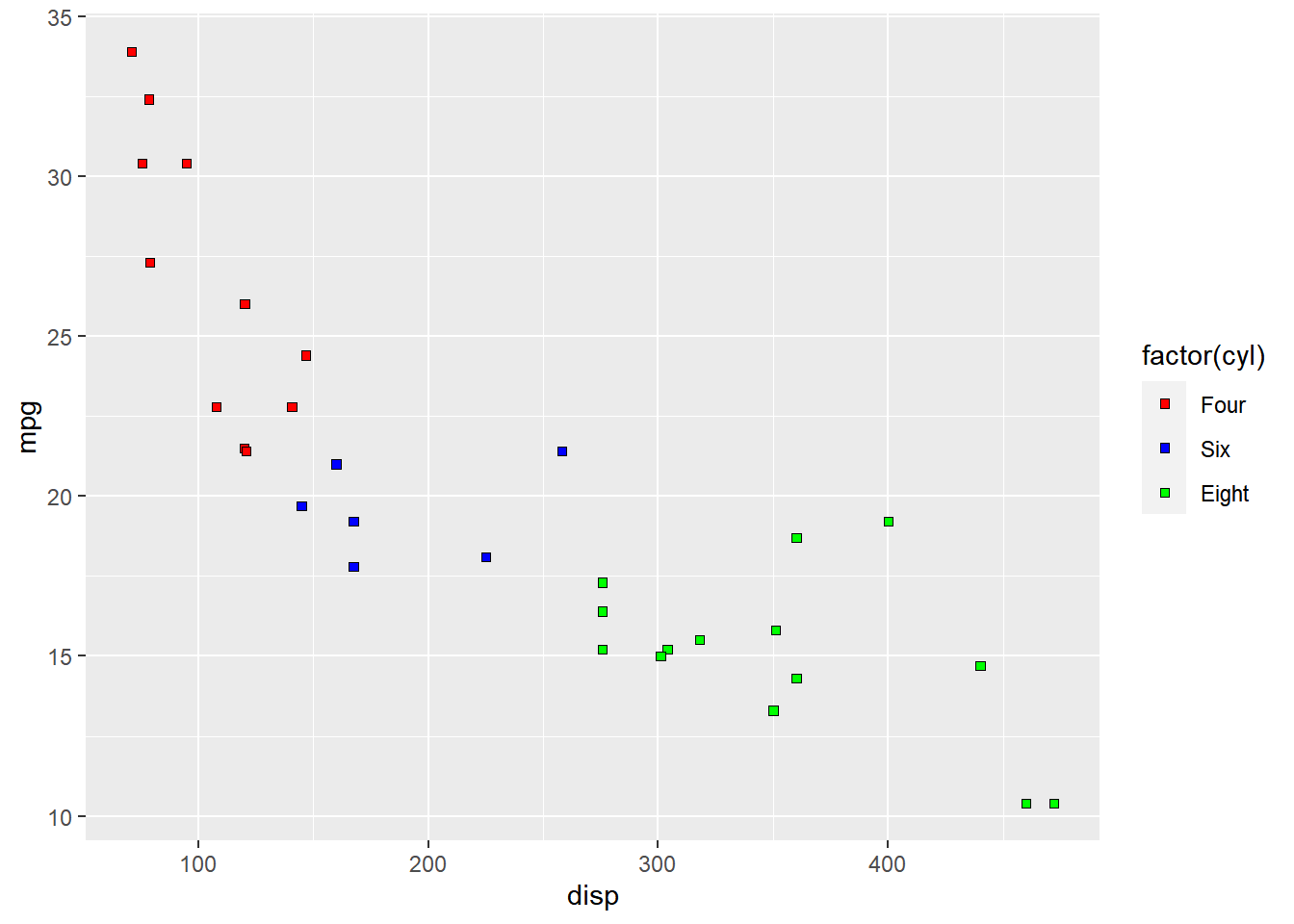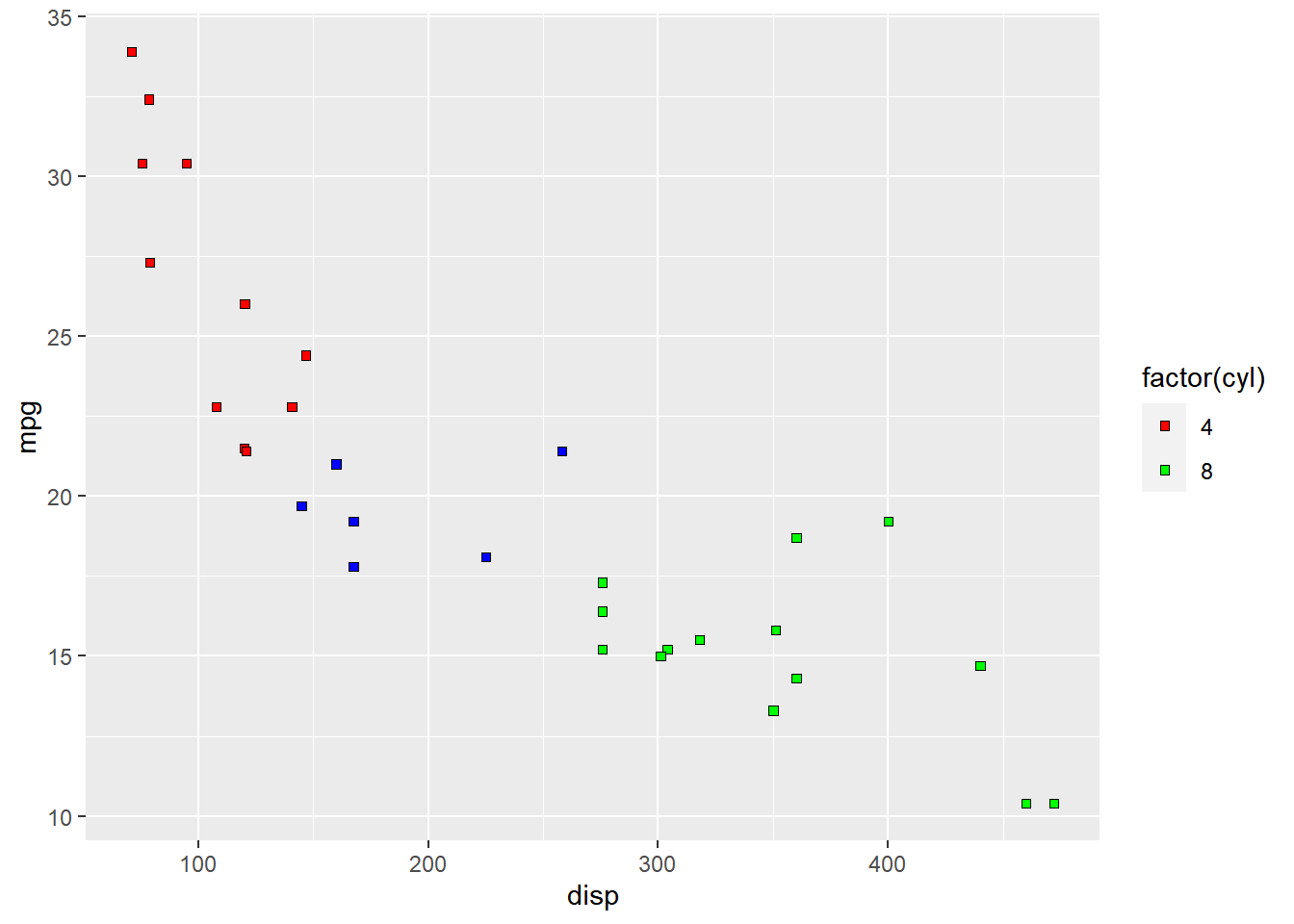# ggplot2: Legend – Part 2

February 23, 2018
By

Want to share your content on R-bloggers? click here if you have a blog, or here if you don't.

### Introduction

This is the 14th post in the series Elegant Data Visualization with ggplot2.
In the previous post, we learnt how to modify the legend of plots when
aesthetics are mapped to variables. In this post, we will continue to explore
different ways to modify/customize the legend of plots.

Specifically, we will learn to modify the following using `scale_fill_manual()`
when `fill` is mapped to categorical variables:

• title
• breaks
• limits
• labels
• values

### Libraries, Code & Data

We will use the following libraries in this post:

All the data sets used in this post can be found here and code can be downloaded from here.

### Plot

displacement and miles per gallon from the mtcars data set. We will map `fill`
to the `cyl` variable.

``````ggplot(mtcars) +
geom_point(aes(disp, mpg, fill = factor(cyl)), shape = 22)``````As you can see, the legend acts as a guide for the `color` aesthetic. Now, let
us learn to modify the different aspects of the legend.

### Title

The title of the legend (`factor(cyl)`) is not very intuitive. If the user
does not know the underlying data, they will not be able to make any sense out
of it. Let us change it to `Cylinders` using the `name` argument.

``````ggplot(mtcars) +
geom_point(aes(disp, mpg, fill = factor(cyl)), shape = 22) +
scale_fill_manual(name = "Cylinders",
values = c("red", "blue", "green"))``````### Values

To change the default colors in the legend, use the `values` argument and
supply a character vector of color names. The number of colors specified
must be equal to the number of levels in the categorical variable mapped.
In the below example, `cyl` has 3 levels (4, 6, 8) and hence we have specified
3 colors.

``````ggplot(mtcars) +
geom_point(aes(disp, mpg, fill = factor(cyl)), shape = 22) +
scale_fill_manual(values = c("red", "blue", "green"))``````### Labels

The labels in the legend can be modified using the `labels` argument. Let us
change the labels to `Four`, `Six` and `Eight` in the next example. Ensure that
the labels are intuitive and easy to interpret for the end user of the plot.

``````ggplot(mtcars) +
geom_point(aes(disp, mpg, fill = factor(cyl)), shape = 22) +
scale_fill_manual(values = c("red", "blue", "green"),
labels = c('Four', 'Six', 'Eight'))``````### Limits

Let us assume that we want to modify the data to be displayed i.e. instead of
examining the relationship between mileage and displacement for all cars, we
desire to look at only cars with at least 6 cylinders. One way to approach this
would be to filter the data using `filter` from dplyr and then visualize it.
Instead, we will use the `limits` argument and filter the data for visualization.

``````ggplot(mtcars) +
geom_point(aes(disp, mpg, fill = factor(cyl)), shape = 22) +
scale_fill_manual(values = c("red", "blue", "green"),
limits = c(6, 8))``````As you can see above, `ggplot2` returns a warning message indicating data related
to 4 cylinders has been dropped. If you observe the legend, it now represents
only 4 and 6 cylinders.

### Breaks

When there are large number of levels in the mapped variable, you may not
want the labels in the legend to represent all of them. In such cases, we can
use the breaks argument and specify the labels to be used. In the below case,
we use the `breaks` argument to ensure that the labels in legend represent
two levels (4, 8) of the mapped variable.

``````ggplot(mtcars) +
geom_point(aes(disp, mpg, fill = factor(cyl)), shape = 22) +
scale_fill_manual(values = c("red", "blue", "green"),
breaks = c(4, 8))``````### Putting it all together…

``````ggplot(mtcars) +
geom_point(aes(disp, mpg, fill = factor(cyl)), shape = 22) +
scale_fill_manual(name = "Cylinders", values = c("red", "blue", "green"),
labels = c('Four', 'Six', 'Eight'), limits = c(4, 6, 8), breaks = c(4, 6, 8))``````### Summary

In this post, we learnt to modify the following aspects of legends:

• title
• breaks
• limits
• labels
• values

### Up Next..

In the next post, we will learn to modify the legend when `shape` is mapped to categorical variables.

R-bloggers.com offers daily e-mail updates about R news and tutorials about learning R and many other topics. Click here if you're looking to post or find an R/data-science job.
Want to share your content on R-bloggers? click here if you have a blog, or here if you don't.